﻿ 快速入门 | 篇十九：正运动技术运动控制器多轴同步与电子凸轮指令简介

# 快速入门 | 篇十九：正运动技术运动控制器多轴同步与电子凸轮指令简介

• 关键词：正运动技术,运动控制器,电子凸轮指令
• 摘要：今天，正运动技术来分享一下，运动控制器多轴同步与电子凸轮指令。这一章节，我们主要分享运动叠加、电子齿轮、电子齿轮2和连接速度等多轴同步指令，以及凸轮表运动、跟随凸轮表运动、自动凸轮和自动凸轮2等凸轮运动指令。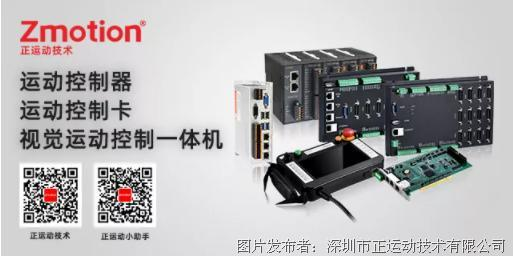1.电脑1台，安装ZDevelop V3.10及以上版本软件；

2.控制器1个；

3.24V直流电源1个；

4.驱动器、电机若干；

5.控制器接线端子若干；

6.网线若干；

7.连接线若干；

8.输入输出设备、扩展模块、人机界面等根据实际需求选择。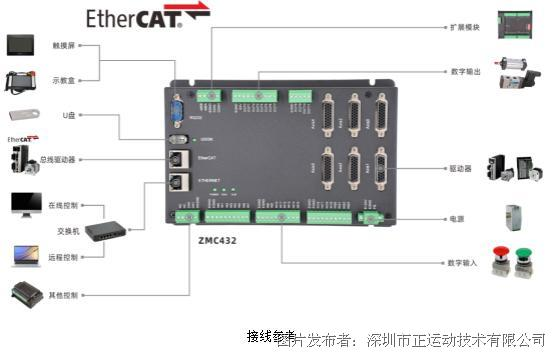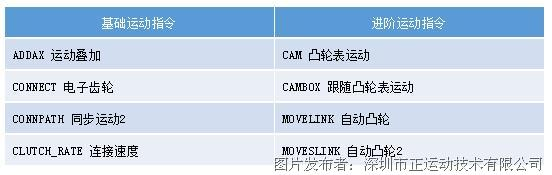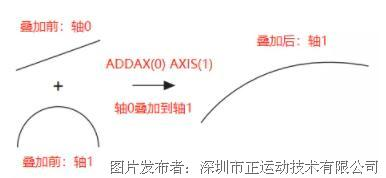RAPIDSTOP(2)

WAIT  IDLE

BASE(0,1)

DPOS=0,0

ATYPE=1,1

UNITS=100,100     '脉冲比例为1:1

SPEED=100,100

ACCEL=1000,1000

DECEL=1000,1000

TRIGGER

MOVE(200)  AXIS(0)

MOVE(-100)  AXIS(1)

WAIT IDLE             '等待运行完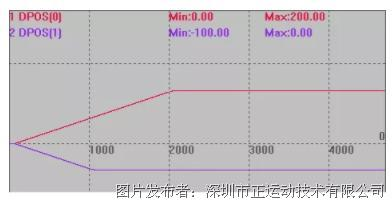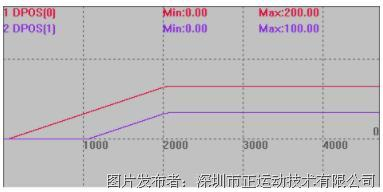2CONNECT --电子齿轮

RAPIDSTOP(2)

WAIT  IDLE(0)

WAIT  IDLE(1)

BASE(0,1)

ATYPE=1,1

UNITS=10,100

DPOS=0,0

SPEED=100,100

ACCEL=1000,1000

DECEL=1000,1000

TRIGGER              '自动触发示波器

MOVE(100)  AXIS(1)         '轴1运动100，此时轴0不动

WAIT IDLE(1)                      '上一段运动不连接

CONNECT(1,1)  AXIS(0)    '轴0连接到轴1，比例为1

MOVE(100)  AXIS(1)          '轴1运动100，轴0运动1000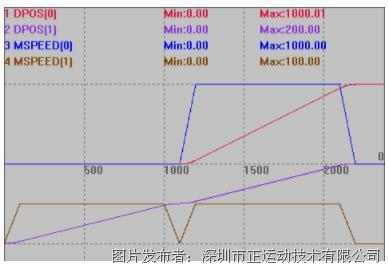RAPIDSTOP(2)

WAIT  IDLE(0)

WAIT  IDLE(1)

BASE(0,1)

ATYPE=1,1

UNITS=10,100

DPOS=0,0

SPEED=100,100

ACCEL=1000,1000

DECEL=1000,1000

TRIGGER              '自动触发示波器

MOVE(100)  AXIS(1)         '轴1运动100，此时轴0不动

WAIT IDLE(1)                      '上一段运动不连接

CONNECT(0.5,1)  AXIS(0)    '轴0连接到轴1，比例为0.5

MOVE(100)  AXIS(1)          '轴1运动100，轴0运动1000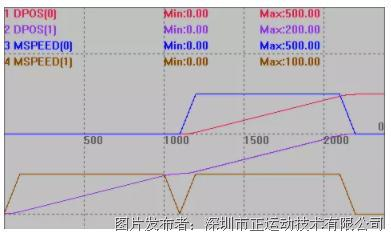3CONNPATH -- 电子齿轮2

CONNPATH与CONNECT的区别：CONNECT连接的是单个轴的目标位置。CONNPATH是连接的是插补轴的矢量长度，此时需要连接在插补运动的主轴上，连接到插补从轴上无法跟随插补运动。例如跟踪XY轴插补的的矢量长度变化，而不是跟踪单独的X轴或者Y轴。

CONNPATH连接到单个轴的运动的效果与CONNECT相同。

RAPIDSTOP(2)

WAIT  IDLE(0)

WAIT  IDLE(1)

WAIT  IDLE(2)

BASE(0,1,2)

DPOS=0,0,0

ATYPE=1,1,1

UNITS=100,100,100

SPEED=100,100,100

ACCEL=1000,1000,1000

DECEL=1000,1000,1000

TRIGGER                  '自动触发示波器

CONNPATH(1,0)  AXIS(2)   '轴2连接到插补主轴轴0，比例为1

MOVE(100,100)            '轴1轴0插补运动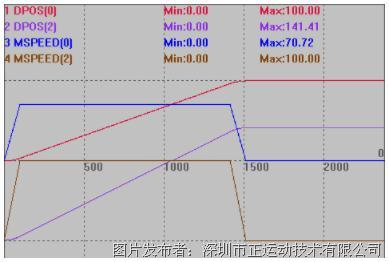RAPIDSTOP(2)

WAIT  IDLE(0)

WAIT  IDLE(1)

WAIT  IDLE(2)

BASE(0,1,2)

DPOS=0,0,0

ATYPE=1,1,1

UNITS=100,100,100

SPEED=100,100,100

ACCEL=1000,1000,1000

DECEL=1000,1000,1000

TRIGGER                  '自动触发示波器

CONNECT(1,0)  AXIS(2)   '轴2连接到插补主轴轴0，比例为1

MOVE(100,100)            '轴1轴0插补运动

同样的例程，CONNECT只能连接到单个轴运动。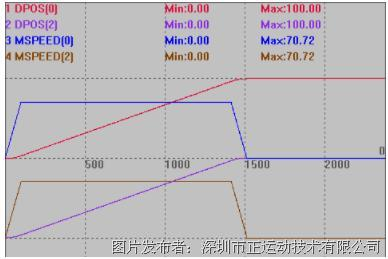RAPIDSTOP(2)

WAIT  IDLE(0)

WAIT  IDLE(1)

WAIT  IDLE(2)

BASE(0,1,2)

DPOS=0,0,0

ATYPE=1,1,1

UNITS=100,100,100

SPEED=100,100,100

ACCEL=1000,1000,1000

DECEL=1000,1000,1000

TRIGGER                  '自动触发示波器

CONNPATH(2,1)  AXIS(2)      '轴2连接到轴1，比例为2

MOVE(100,100)         '轴1轴0插补运动

MOVE(100)  AXIS(1)     '轴1运动100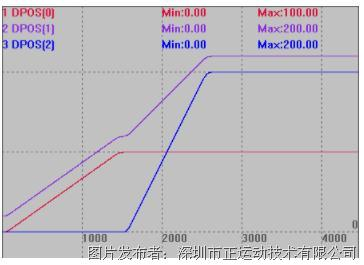4CLUTCH_RATE -- 连接速度

CONNECT连接的速度，默认值1000000，用于定义连结率从0到设置倍率的改变时间，ratio/秒。

CONNPATH同样适用。

RAPIDSTOP(2)

WAIT  IDLE(0)

WAIT  IDLE(1)

BASE(0,1)

ATYPE=1,1

DPOS=0,0

UNITS=100,100

SPEED=100,100

ACCEL=1000,1000

DECEL=1000,1000

CLUTCH_RATE=1            '设置连接速度，倍率/s

TRIGGER                    '自动触发示波器

CONNECT(2,1)  AXIS(0)        '连接倍率为2，需要2秒建立连接

MOVE(200)  AXIS(1)            '运动轴1，轴0跟随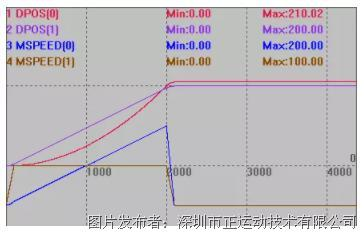RAPIDSTOP(2)

WAIT  IDLE(0)

WAIT  IDLE(1)

BASE(0,1)

ATYPE=1,1

DPOS=0,0

UNITS=100,100

SPEED=100,100

ACCEL=1000,1000

DECEL=1000,1000

CLUTCH_RATE=3            '设置连接速度，倍率/s

TRIGGER                    '自动触发示波器

CONNECT(2,1)  AXIS(0)        '连接倍率为2，需要2秒建立连接

MOVE(200)  AXIS(1)            '运动轴1，轴0跟随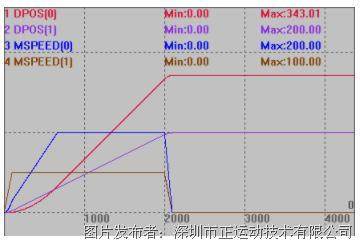RAPIDSTOP(2)

WAIT  IDLE(0)

WAIT  IDLE(1)

BASE(0,1)

ATYPE=1,1

DPOS=0,0

UNITS=100,100

SPEED=100,100

ACCEL=1000,1000

DECEL=1000,1000

CLUTCH_RATE=1000000            '设置连接速度，倍率/s

TRIGGER                    '自动触发示波器

CONNECT(2,1)  AXIS(0)        '连接倍率为2，需要2秒建立连接

MOVE(200)  AXIS(1)            '运动轴1，轴0跟随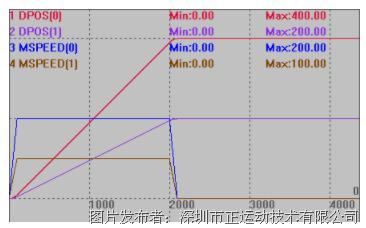RAPIDSTOP(2)

WAIT  IDLE(0)

WAIT  IDLE(1)

BASE(0,1)

ATYPE=1,1

DPOS=0,0

UNITS=100,100

SPEED=100,100

ACCEL=1000,1000

DECEL=1000,1000

CLUTCH_RATE=0            '设置连接速度，倍率/s

TRIGGER                    '自动触发示波器

CONNECT(2,1)  AXIS(0)        '连接倍率为2，需要2秒建立连接

MOVE(200)  AXIS(1)            '运动轴1，轴0跟随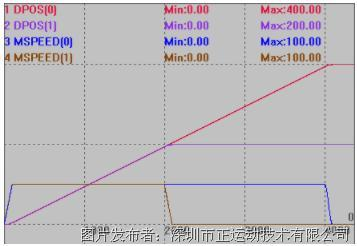5CAM --凸轮表运动

start point：起始点TABLE编号，存储第一个点的位置。

end point：结束点TABLE编号。

table multiplier：位置乘以这个比例，一般设为脉冲当量值，TABLE数据*table multiplier值=实际发出的脉冲数。

distance：参考运动的距离，总时间=distance/轴speed。

TBALE数据需要手动设置，第一个数据为引导点，建议设为0。

RAPIDSTOP(2)

WAIT IDLE(0)

BASE(0) '选择第0轴

ATYPE=1  '脉冲方式步进或伺服

DPOS = 0

UNITS = 100'脉冲当量

SPEED = 200

ACCEL = 2000

DECEL = 2000

'计算TABLE的数据

DIM deg, rad, x, stepdeg

stepdeg = 2     '可以通过这个来修改段数，段数越多速度越平稳

FOR deg=0 TO 360 STEP stepdeg

rad = deg * 2 * PI/360   '转换为弧度

X = deg * 25 + 10000 * (1-COS(rad)) '计算每小段位移

TABLE(deg/stepdeg,X)        '存储TABLE

TRACE deg/stepdeg,X

NEXT deg

TRIGGER   '触发示波器采样

WHILE 1        '循环运动

CAM(0, 360/stepdeg, 0.1, 300)    '虚拟跟踪总长度300

WAIT UNTIL IDLE    '等待运动停止

WEND

END

TABLE存储的凸轮表参数：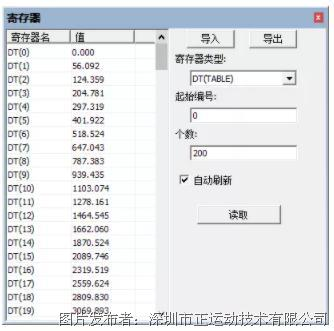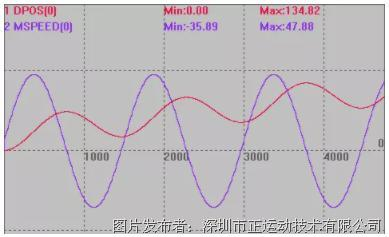DIM  num_p,scale,m,t   '变量定义

num_p=100

scale=500

FOR p=0 TO num_p   'table存储凸轮表运动参数

TABLE(p,((-SIN(PI*2*p/num_p)/(PI*2))+p/num_p)*scale)

NEXT

RAPIDSTOP(2)

WAIT  IDLE(0)

WAIT  IDLE(1)

BASE(0)      '选择轴0

DEFPOS(0)

UNITS=500

SPEED=1000

ACCEL=1000000

DECEL=1000000

TRIGGER

m=10        '代表距离的倍数

t=0.3       '运行时间

SPEED=1000

CAM(0,100,m,SPEED*t)

WAIT IDLE

m=10

t=0.3

SPEED=1000

CAM(0,100,-m,SPEED*t)

WAIT IDLE

m=10

t=0.2

SPEED=500

CAM(0,100,m,SPEED*t)

WAIT IDLE

m=10

t=0.2

SPEED=500

CAM(0,100,-m,SPEED*t)

WAIT IDLE

m=20

t=0.3

SPEED=1000

CAM(0,100,m,SPEED*t)

WAIT IDLE

m=20

t=0.5

SPEED=500

CAM(0,100,-m,SPEED*t)

WAIT IDLE

TABLE存储的凸轮表参数：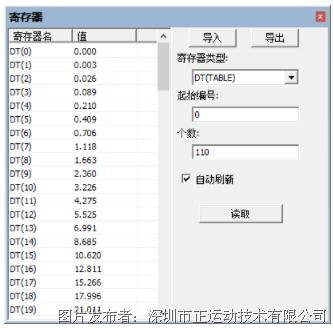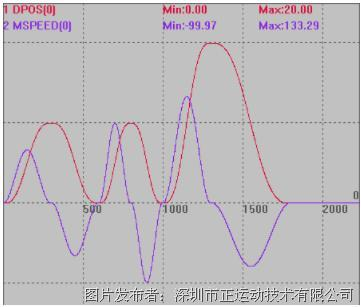6CAMBOX --凸轮表运动

start point：起始点TABLE编号，存储第一个点的位置。

end point：结束点TABLE编号。

table multiplier：位置乘以这个比例，一般设为脉冲当量值，TABLE数据*table multiplier值=实际发出的脉冲数。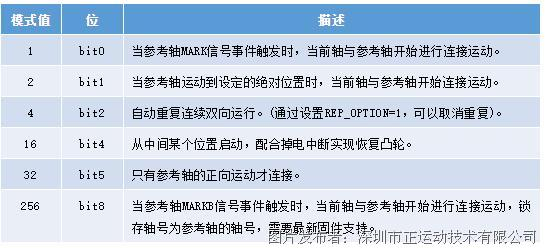TBALE数据需要手动设置，第一个数据为引导点，建议设为0。

RAPIDSTOP(2)

WAIT  IDLE(0)

WAIT  IDLE(1)

BASE(0,1)      '选择轴号

ATYPE=1,1     '脉冲方式步进或伺服

DPOS = 0,0

UNITS = 100,100  '脉冲当量

SPEED = 200,200

ACCEL = 2000,2000

DECEL = 2000,2000

FOR i=0 TO 100 STEP 1

rad = i* 2 * PI/100   '转换为弧度

x = 1000 * (1-COS(rad))

TABLE(i,x)        '存储TABLE

NEXT i

TRIGGER              '自动触发示波器

CAMBOX(0,100, 100, 2000, 1,2,100)  AXIS(0) '参考轴轴1运动到100位置时，跟随轴轴0启动

VMOVE(1)  AXIS(1)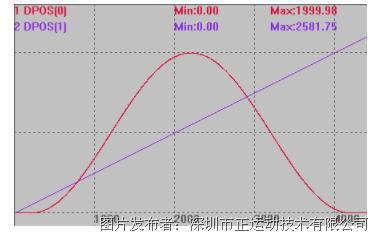RAPIDSTOP(2)

WAIT  IDLE(0)

WAIT  IDLE(1)

BASE(0,1)      '选择轴号

ATYPE=1,1     '脉冲方式步进或伺服

DPOS = 0,0

UNITS = 100,100  '脉冲当量

SPEED = 200,200

ACCEL = 2000,2000

DECEL = 2000,2000

FOR i=0 TO 100 STEP 1

rad = i* 2 * PI/100   '转换为弧度

x = 1000 * (1-COS(rad))

TABLE(i,x)        '存储TABLE

NEXT i

TRIGGER              '自动触发示波器

CAMBOX(0,100, 100, 2000, 1,2,100)  AXIS(0) '参考轴轴1运动到100位置时，跟随轴轴0启动

MOVE(1500)  AXIS(1)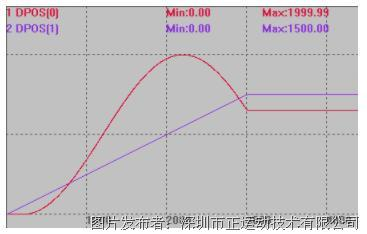distance：从连接开始到结束，跟随轴移动的距离，此参数可正可负，为正数正方向跟随，为负数负方向跟随，采用units单位。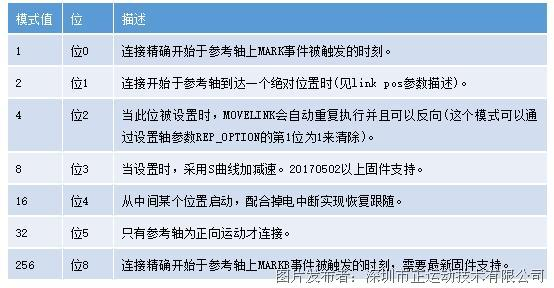RAPIDSTOP(2)

WAIT  IDLE(0)

WAIT  IDLE(1)

BASE(0,1)    '轴0为跟随轴，轴1为参考轴

UNITS=100,100

ATYPE=1,1

DPOS=0,0

SPEED=100,100

ACCEL=2000,2000

DECEL=2000,2000

TRIGGER              '自动触发示波器

MOVE(150)  AXIS(1)      '轴1运动150，轴0跟随轴1运动完100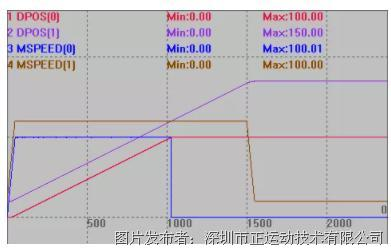RAPIDSTOP(2)

WAIT  IDLE(0)

WAIT  IDLE(1)

BASE(0,1)

UNITS=100000,100000

ATYPE=1,1

DPOS=0,0

SPEED=1,1    '型材运行速度1m/s,60m/min

ACCEL=2,2

DECEL=2,2

MERGE=on,on

MOVE_OP2(0,on,1000)     '刀具下剪，1s后回升(时间要计算好)

TRIGGER         '自动触发示波器

VMOVE(1) AXIS(1)    '型材持续运动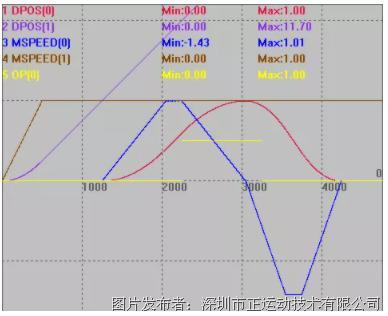RAPIDSTOP(2)

WAIT  IDLE(0)

WAIT  IDLE(1)

BASE(0,1)

UNITS=100000,100000

ATYPE=1,1

DPOS=0,0

SPEED=1,1      ''型材运行速度1m/s,60m/min

ACCEL=2,2

DECEL=2,2

SRAMP=200,200     '设置S曲线时间

OP(0,OFF)

MOVE_OP2(0,on,1000)   '刀具下剪，1s后回升(时间要计算好)

TRIGGER         '自动触发示波器

VMOVE(1) AXIS(1)    '型材持续运动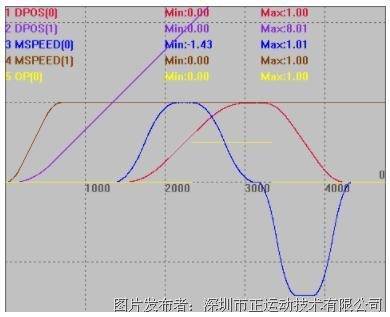distance：从连接开始到结束，跟随轴移动的距离，此参数可正可负，为正数正方向跟随，为负数负方向跟随，采用units单位。

start sp：启动时跟随轴和参考轴的速度比例，units/units单位，负数表示跟随轴负向运动。

end sp：结束时跟随轴和参考轴的速度比例，units/units单位, 负数表示跟随轴负向运动 注：当start sp = end sp = distance/link dist时，匀速运动。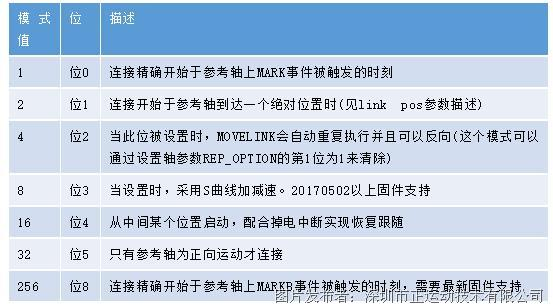RAPIDSTOP(2)

WAIT  IDLE(0)

WAIT  IDLE(1)

BASE(0,1)

UNITS=10000,10000

ATYPE=1,1

DPOS=0,0

SPEED=1,1      '型材运行速度1m/s,60m/min

ACCEL=2,2

DECEL=2,2

SRAMP=200,200

OP(0,OFF)

TRIGGER         '自动触发示波器

VMOVE(1) AXIS(1)    '型材持续运动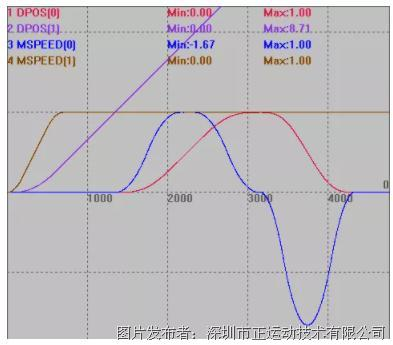RAPIDSTOP(2)

WAIT  IDLE(0)

WAIT  IDLE(1)

BASE(0,1)

UNITS=10000,10000

ATYPE=1,1

DPOS=0,0

SPEED=1,1      '型材运行速度1m/s,60m/min

ACCEL=2,2

DECEL=2,2

SRAMP=0,0

OP(0,OFF)

TRIGGER         '自动触发示波器

VMOVE(1) AXIS(1)    '型材持续运动

S曲线只针对型材，工作台速度不管设不设置曲线自动平滑。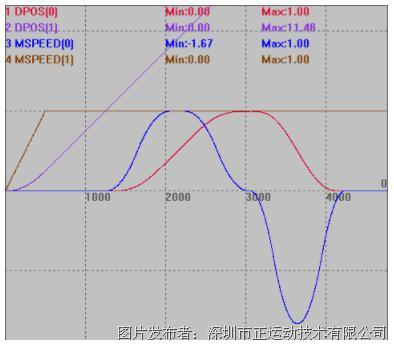• 1494 2020/9/30
• 2099 2021/6/23
• 1111 2022/2/21
• 1486 2021/8/24
• 1580 2021/10/29
• 1607 2020/9/25